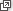### Discrete and continuous growth

We have seen many examples of a quantity $$x$$ which continuously grows (or decays) over time by an amount proportional to $$x$$, with continuous growth rate $$k$$. Such an $$x$$ obeys a differential equation with an exponential solution:

$\dfrac{dx}{dt} = kx \quad \iff \quad x(t) = C e^{kt}, \text{ where $$C$$ is a constant.}$

In discussing interest rates, however, we saw an example of a quantity $$x$$ which grows in discrete steps by an amount proportional to $$x$$. Such an $$x$$ obeys a difference equation with an exponential solution:

$x(t+1) - x(t) = r \, x(t) \quad \iff \quad x(t) = (1+r)^t \, x(0).$

Thinking of this difference equation as $$\Delta x = rx$$, by analogy with the continuous case we call $$r$$ the discrete growth rate. At each step, $$x$$ is multiplied by $$1+r$$, and $$x(t)$$ is obtained from $$x(0)$$ by $$t$$ such multiplications. As we have derived it, this equation only holds for integers $$t$$. However, if we imagine it is valid for all real $$t$$, then $$x(t)$$ is a continuous function. Rewriting using log laws,

$x(t) = e^{t \, \log_e (1+r)} \, x(0).$

We may then differentiate, noting that $$x(0)$$ is a constant:

\begin{align*} \dfrac{dx(t)}{dt} &= \log_e (1+r) \, e^{t \, \log_e (1+r)} \, x(0) \\ &= \log_e (1+r) \, x(t). \end{align*}

We conclude that the discrete growth rate $$r$$ corresponds to a continuous growth rate of

$k = \log_e (1+r).$

We saw this fact in exercise 8.

#### Discrete and continuous interest

In the module Exponential and logarithmic functions, we discussed a related concept. We imagined that, instead of paying interest once a year at a rate of $$r$$, a bank might add interest twice a year at the rate $$\dfrac{r}{2}$$, or three times a year at the rate $$\dfrac{r}{3}$$. In general, we considered interest added $$n$$ times a year at the rate of $$\dfrac{r}{n}$$. In this case, each year your money is multiplied by

$\Bigl( 1 + \dfrac{r}{n} \Bigr)^n.$

We saw that, as $$n \to \infty$$, this quantity approaches $$e^r$$:

$\lim_{n \to \infty} \Bigl( 1 + \dfrac{r}{n} \Bigr)^n = e^r.$

So the quantity $$x(t)$$ of money is multiplied by $$e^r$$ each year, and

$x(t) = e^{rt} \, x(0).$

Therefore

\begin{align*} \dfrac{dx(t)}{dt} &= r \, e^{rt} \, x(0) \\ &= r \, x(t), \end{align*}

and the continuous growth rate is $$r$$.

Hence, if we take a discrete growth rate (or interest rate) of $$r$$, it corresponds to a continuous growth rate of $$\log_e (1+r)$$. But if that discrete growth is divided into an interest rate of $$\dfrac{r}{n}$$, applied $$n$$ times as often, in the limit we obtain continuously compounded interest at a continuous growth rate of $$r$$.

#### Discrete and continuous population growth

The distinction between discrete and continuous growth also applies to populations. We have seen models of exponential population growth with constant continuous growth rates — usually unrealistic models.

However, in a non-technical context, the 'growth rate' of a population is sometimes taken to mean something like the percentage increase in population from the previous year. We can translate this discrete growth rate into a continuous growth rate, as in the following example.

#### Example

The Australian Bureau of Statistics estimated that in mid-2012 the resident population of Australia was $$22\ 683\ 600$$, an increase of $$1.6\%$$ from the previous year. Assuming (inaccurately) a constant population growth rate $$k$$, find $$k$$ to three significant figures.

#### Solution

With an increase of $$1.6\%$$ in a year, the discrete growth rate is $$r = 0.016$$. Thus, as discussed in this section, the corresponding continuous growth rate is

\begin{align*} k &= \log_e (1+r) \\ &= \log_e 1.016 \\ &\approx 0.0159 = 1.59\% \ \ \text{(to three significant figures)}. \end{align*}

References

• Data from Australian Bureau of Statistics• Since 1973, the percentage increase in population per year in Australia has varied between $$1.0\%$$ and $$1.8\%$$ (Australian Bureau of Statistics, Australian Historical Population Statistics, 2008, 3105.0.65.001).

Note that in this example the discrete and continuous growth rates are very close. This is because $$\log_e (1+x) \approx x$$ for $$x$$ close to 0. The two growth rates are close when they are small, but not when they are large.

Exercise 9

Return to our pheasants from exercise 4, which for a few years had a constant continuous growth rate of about 1. What was their discrete growth rate?

Next page - Links forward - Logistic growth